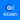# Practice Quiz in Op-Amp Applications Part 2

(Last Updated On: February 3, 2020)

This is the Online Practice Quiz in Op-Amp Applications Part 2 from the book, Electronic Devices and Circuit Theory 10th Edition by Robert L. Boylestad. If you are looking for a reviewer in Electronics Engineering this will definitely help. I can assure you that this will be a great help in reviewing the book in preparation for your Board Exam. Make sure to familiarize each and every questions to increase the chance of passing the ECE Board Exam.

### Continue Part II of the Online Practice Quiz

#### Quiz in Op-Amp Applications

Question 11. Determine the output voltage for this circuit with a sinusoidal input of 2.5 mV.A. –0.25 V

B. –0.125 V

C. 0.25 V

D. 0.125 V

Question 12. Calculate the cutoff frequencies of a bandpass filter with R1 = R2 = 5 kΩ and C1 = C2 = 0.1 μF.

A. fOL = 318.3 Hz, fOH = 318.3 Hz

B. fOL = 636.6 Hz, fOH = 636.6 Hz

C. fOL = 318.3 Hz, fOH = 636.6 Hz

D. fOL = 636.6 Hz, fOH = 318.3 Hz

Question 13. Determine the output voltage when V1 = –V2 = 1 V.A. 0 V

B. –2 V

C. 1 V

D. 2 V

Question 14. Calculate the output voltage.A. –6.00 mV

B. 6.0 mV

C. 6.12 mV

D. –6.12 mV

Question 15. Determine the output voltage when V1 = –V2 = –1 V.A. 0 V

B. –2 V

C. 1 V

D. 2 V

Question 16. Calculate the output voltage if V1 = –0.2 V and V2 = 0 V.A. 0 V

B. –6.6 V

C. –4 V

D. 2 V

Question 17. Calculate IL for this circuit.A. 3 mA

B. 4 mA

C. 5 mA

D. 6 mA

Question 18. Calculate the output voltage.A. 3.02 V

B. 2.03 V

C. 1.78 V

D. 1.50 V

Question 19. Calculate the output voltage if V1 = V2 = 0.15 V.A. 0 V

B. 4.65 V

C. 6.45 V

D. –6.45 V

Question 20. Calculate the output voltage if V1 = 33 mV and V2 = 02 mV.A. 0 V

B. –6.6 V

C. –4 V

D. 2 V

### More Practice Quiz in Op-Amp Applications

Practice Quiz Part 1

Practice Quiz Part 2

Practice Quiz Part 3

Practice Quiz Part 4

Practice Quiz Part 5

Practice Quiz Part 6

### See: Complete List of Practice Quizzes

Note: After taking this particular quiz, you can proceed to check all the topics.

Help Me Makes a Difference!

 P inoyBIX educates thousands of reviewers/students a day in preparation for their board examinations. Also provides professionals with materials for their lectures and practice exams. Help me go forward with the same spirit. “Will you make a small gift today via GCASH?”+63 966 459 6474 Option 1 : \$1 USD Option 2 : \$3 USD Option 3 : \$5 USD Option 4 : \$10 USD Option 5 : \$25 USD Option 6 : \$50 USD Option 7 : \$100 USD Option 8 : Other Amount© 2014 PinoyBIX Engineering. © 2019 All Rights Reserved | How to Donate? |